Author: Oscar Cronquist Article last updated on May 02, 2019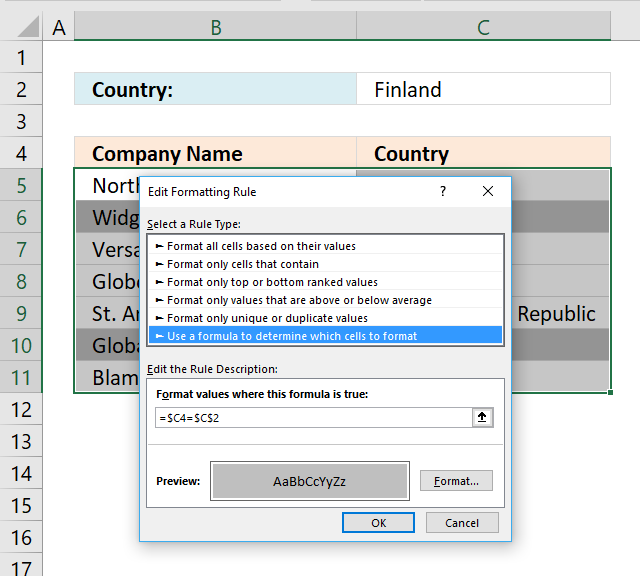In this post I am going to try to explain formula basics in conditional formatting. It is really good if you have some knowledge about absolute and relative cell references but it is not necessary. I think the examples shown here explains a lot when it comes to cell referencing.

Conditional formatting is extremely volatile and is even recalcualted every time you scroll the sheet. Remember this if you already work with cpu instensive workbooks.

When creating conditional formatting formulas, I think you will be using mostly comparison operators (=<>) but you can also use functions. Functions I recommend: COUNTIF(), COUNTIFS(), SEARCH(), FIND()

### How to create conditional formatting

1. Select a cell range where you want to apply conditional formatting.
2. Go to "Home" tab
3. Press with left mouse button on "Conditional Formatting" button
4. Press with left mouse button on "New Rule.."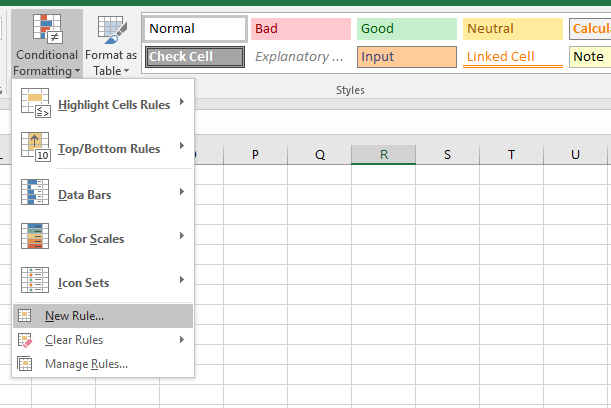5. Press with left mouse button on "Use a formula to determine which cells to format:"
6. Type formula in "Format values where this formula is true:"
7. Press with left mouse button on "Format..." button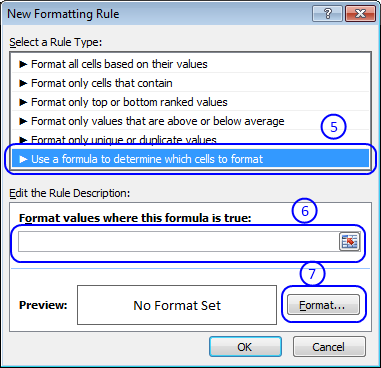8. Choose formatting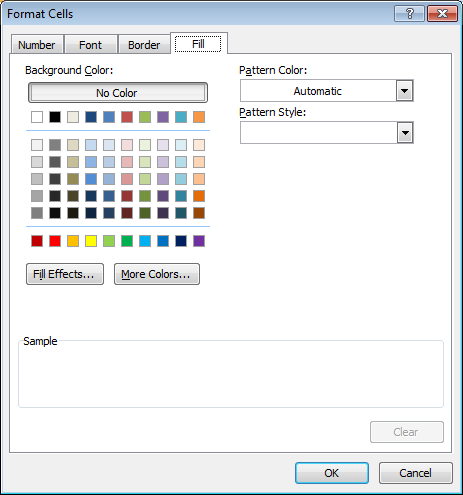9. Press with left mouse button on OK
10. Press with left mouse button on OK

### Your first conditional formatting formula

Let´s create your first conditional formatting formula. Here is a table with some random data.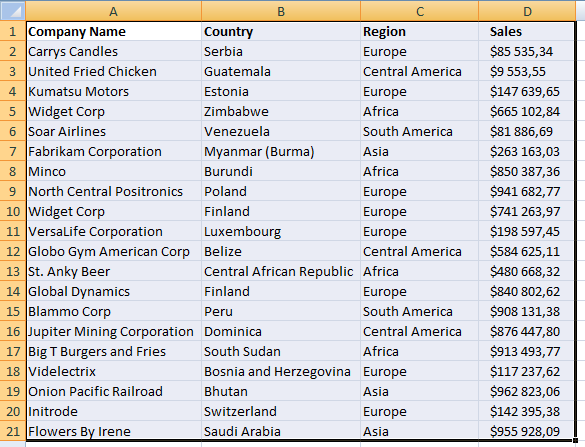Select cell range A1: D21 Repeat above steps and use this formula:

=A1="Poland"

I used a grey formatting color.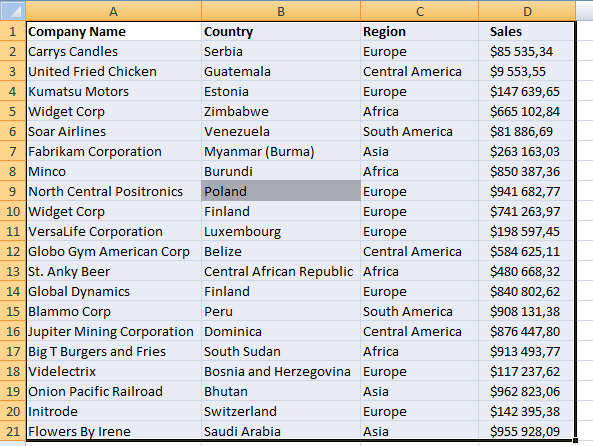=A1="Poland"

A1 is a cell reference to the uppermost, leftmost cell in your selected cell range. Relative cell reference changes depending on which cell is calculated. Using cell A1 in the conditional formatting formula, applied to cell range A1:D21 makes it compare each cell in the cell range to the value "Poland".

Example 1,

In cell A1:

Conditional formatting formula:

=A1="Poland"

becomes

="Company Name"="Poland"

and returns False. Cell A1 is NOT formatted.

Example 2,

In cell B9:

The conditional formatting formula changes automatically to:

=B9="Poland"

becomes

="Poland"="Poland"

and returns True. Cell B9 is formatted.

Which cells are highighted if the conditional formatting is =A2="Poland"?

### Format entire row

Let´s say you are interested in highlighting rows where cell value in column B is equal to "Poland".

Conditional formatting formula:

=\$B1="Poland"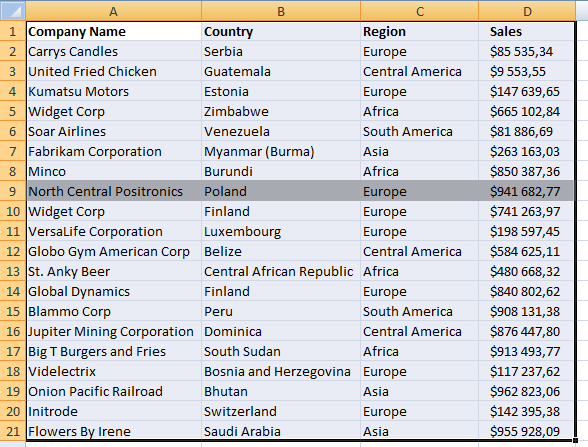Example 1,

In cell A1:

Conditional formatting formula:

=\$B1="Poland"

becomes

="Country"="Poland"

and returns False. Cell A1 is NOT formatted.

Example 2,

In cell A9:

The conditional formatting formula changes automatically to:

=\$B9="Poland"

becomes

="Poland"="Poland"
and returns True. Cell A9 is formatted. In fact, the entire row is highlighted. The \$ makes the cell reference "locked" to column B.

### Compare a cell value to a column

Every time you want to change the value you are searching for you must first select the cell range, change the conditional formatting formula and then press OK. Boring repetitive actions, but if you change the value in the conditional formatting formula to an absolute cell reference things start to become more useful. Let me show you how: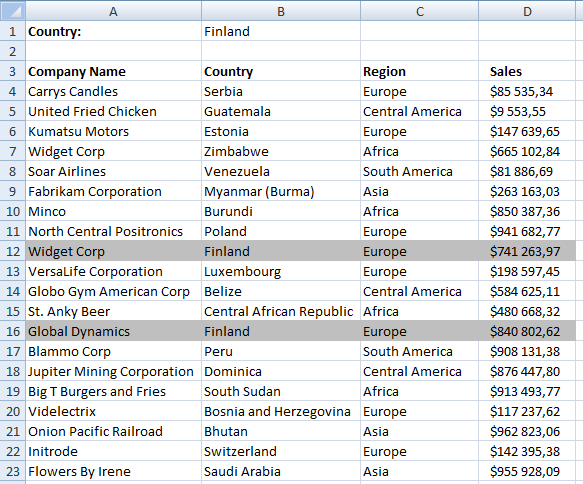Conditional formatting formula:

=\$B4=\$B\$1

Cell value in B1 is compared to every cell value in column B. If the conditional formatting formula returns TRUE the entire row is highlighted.
With the same technique you can highlight an entire column, example: Search with conditional formatting

### Column to column comparisons

Now I am going to show you how to use conditional formatting to easily identify companies with increasing sales.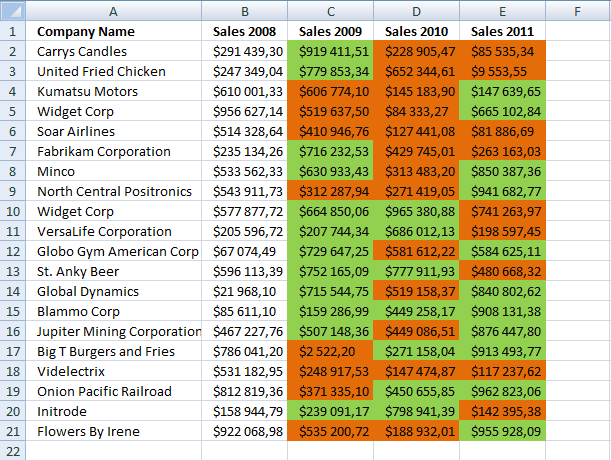Highlight increasing sales years

Conditional formatting formula:

=C2>B2

Highlight decreasing sales years

Conditional formatting formula:

=C2<B2
Remember to use the uppermost leftmost cell reference in the selected cell range when constructing conditional formatting formulas. In this example, I have applied CF to cell range C2:E21 and C2 is the first cell in that range to be compared to the previous year.

### Highlight unique rows

This example demonstrates how to highlight unique distinct rows.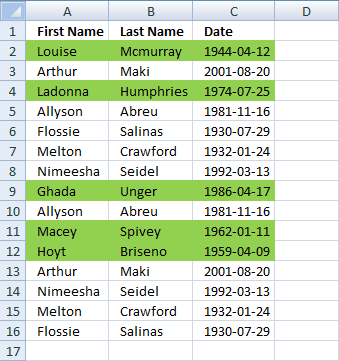Conditional formatting formula:

=COUNTIFS(\$A\$2:\$A\$16, \$A2, \$B\$2:\$B\$16, \$B2, \$C\$2:\$C\$16, \$C2)=1

### Get the Excel fileWorking-with-conditional-formatting.xlsx

Highlight unique distinct values

Highlight duplicates values

Highlight duplicate rows (COUNTIFS function)

Compare two lists of data: Highlight records existing in only one list

Compare two lists of data: Highlight common records

How to create a unique list using conditional formatting

Highlight common values in two lists using conditional formatting

Search with conditional formatting